×#### Thank you for registering.

One of our academic counsellors will contact you within 1 working day.

Click to Chat

1800-1023-196

+91-120-4616500

CART 0

• 0

MY CART (5)

Use Coupon: CART20 and get 20% off on all online Study Material

ITEM
DETAILS
MRP
DISCOUNT
FINAL PRICE
Total Price: Rs.

There are no items in this cart.
Continue Shopping• Complete JEE Main/Advanced Course and Test Series
• OFFERED PRICE: Rs. 15,900
• View Details

```Chapter 6: Graphs of Trigonometric Functions – Exercise 6.1

Graphs of Trigonometric Functions – Exercise 6.1 – Q.1

To obtain the graph of y = 3 sin x we first draw the graph of y = sin x in the interval [0, 2x]. The maximum and minimum values are 3 and - 3 respectively.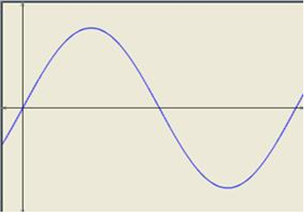We have,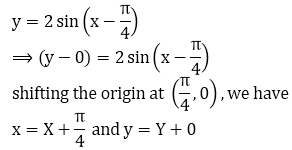Substituting these values in (i), we get

Y = 2sin x

Thus we draw the graph of Y = 2 sin X and shift it by π/4 to the right to get the required graph.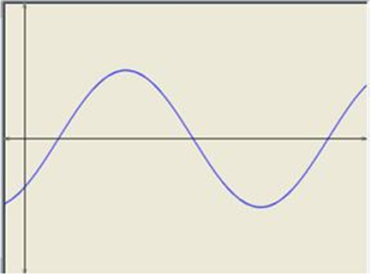We have,Substituting these values in (i), we get

Y = 2sin 2X

Thus we draw the graph of Y = 2 sin 2X and shift it by 1/2 to the right to get the required graph.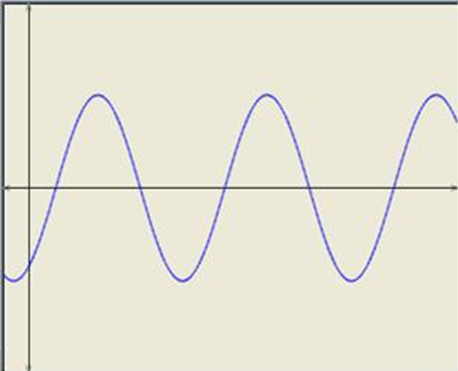We have,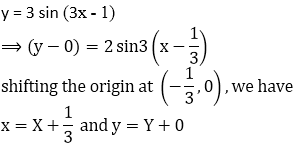Substituting these values in (i), we get

Y = 2sin 3X

Thus we draw the graph of Y = 3 sin 3X and shift it by 1/3 to the right to get the required graph.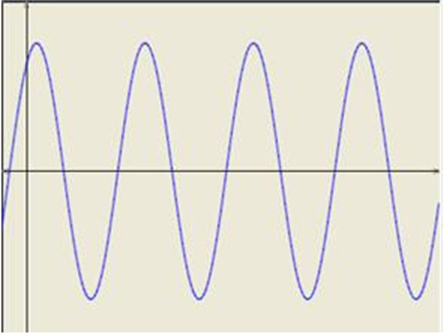We have,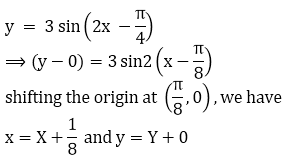Substituting these values in (i), we get

Y = 3 sin 2X

Thus we draw the graph of Y = 3 sin 2X and shift it by 1/8 to the right to get the required graph.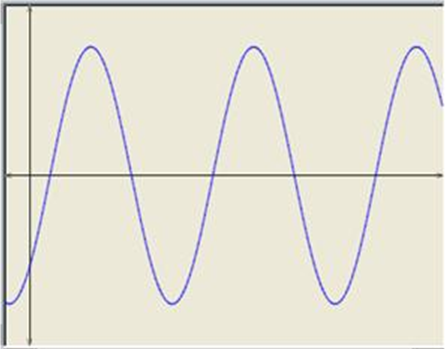Graphs of Trigonometric Functions – Exercise 6.1 – Q.2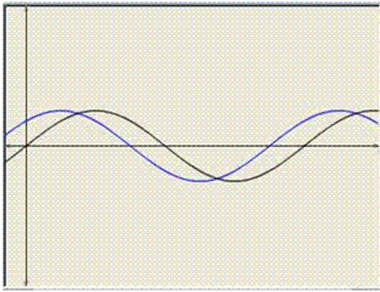We have,Substituting these values in (i), we get

Y = sin X.

Thus we draw the graph of Y = sin X and shift it by π/4 to the right to get the required graph.

To obtain the graph of y = sin 3x we first draw the graph of y = sin x in the interval [0, 2π] and then divide the x-coordinates of the points where it crosses x-axis by 3.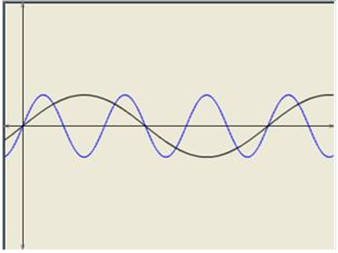```### Course Features

• 728 Video Lectures
• Revision Notes
• Previous Year Papers
• Mind Map
• Study Planner
• NCERT Solutions
• Discussion Forum
• Test paper with Video Solution